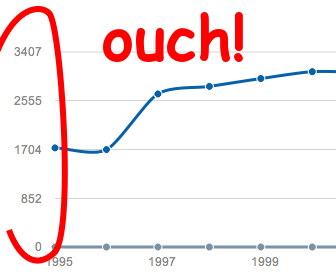# Chart libraries headaches – finding the best grid stepIf you have ever made a chart library in your life, you’ve probably asked yourself how to find the best scale for the grid in order to have nice values to display in the axis.

Most of the time, data ranges are unknown, hence we need to adapt the grid step to provide the best display.

## Check this out

Let’s explain the algorithm…

Any number can be formatted in scientific notation. It is written in the form of A x 10N and is noted AeN.

For instance, 2300 becomes 2.3e3 (because 2300 = 2.3 x 103), 12 becomes 1.2e1, and 0.23 becomes 2.3e-1.

Scientific notation is exactly made for displaying huge or tiny values in a few characters.
We can use the same principle for finding good values for the step scale, we can just keep the pow of 10 part (N) and round the value part (A).

## Magic numbers

But rounding is not enough, I have found that good pattern numbers of step range is those divisible by 2, 5 and 10.

In math term, we need to find a step range sr, where

``````∀ n ∈ |N, ∀ a ∈ {1, 2, 5}, ∃ sr, sr = a x 10^n
``````

This is basically because 2 x 5 = 10 : using a step of 5 we have a 10 modularity every 2 step, and, using a step of 2 we have a 10 modularity every 5 step.

** 2 step:** 0 2 4 6 8 10 12 14 16 18 20
** 5 step:** 0 5 10 15 20 25 30 35 40 45 …
10 step: 0 10 20 30 40 50 60 70 80 90

For any dataset, we need to fallback on the closest step range in all of possible step ranges: … 0.002, 0.02, 0.2, 2, 20, 200, …, … 0.005, 0.05, 0.5, 5, 50, 500, …, and … 0.001, 0.01, 0.1, 1, 10, 100, …,

### Calculate the pow of 10

To get the N value of the A x 10N form, we can use the log of 10:

``````N = Math.log(number) / Math.log(10);
``````

### Calculate the value modulo 10

To get the A value of the A x 10N form, we can just divide the number by 10N:

``````A = number / Math.pow(10, N);
``````

### ‘Rounding’ the number

We know just need to change the value of A and make it more “readable”.
We can map the value as follow:

``````if A ∈ [0, 1.5[ then A becomes 1
if A ∈ [1.5, 3.5[ then A becomes 2
if A ∈ [3.5, 7.5[ then A becomes 5
if A ∈ [7.5, 10[ then A becomes 10
``````

Note that these rules may probably be improved, I would love if someone could improve this (because I use a arithmetic mean approach and it should probably be arithmetic).

## Implementation

### Javascript

Usage example:

``````GridUtils.findNiceRoundStep(xMax, 10);
``````

where xMax is the scale of the axis, and 10 is the desired number of graduation split.

## Conclusion

Finding the best grid step is finally a simple thing to implement but is an essential feature every chart libraries should have.As a generative plotter artist, I use code to generate art (creative coding) and physically create it with pen plotters, which is itself a generative process – each physical plot is a unique variant. I love dualities, like digital vs analog physical, abstract vs figurative, orthogonal vs polar, photo vs noise,...